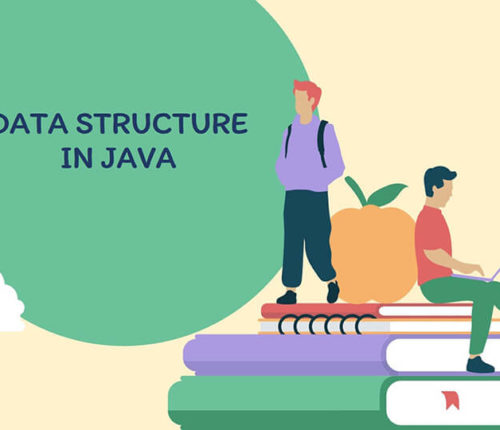# Find subarrays with given sum in an array.

If you want to practice data structure and algorithm programs, you can go through Java coding interview questions.

In this post, we will see how to find subarrays with given sum in an array.

## Problem

Given an Array of non negative Integers and a number. You need to print all the starting and ending indices of Subarrays having their sum equal to the given integer.
For Example :-

Input-int[] arr = {2, 3, 6, 4, 9, 0, 11};
int num = 9
Output-

starting index : 1, Ending index : 2
starting index : 5, Ending index : 5
starting index : 5, Ending index : 6

Explanation :
[3, 6] ,
[9,0] These all are the subarrays with their sum equal to 9.

## Solution

Naive Method:
The basic brute force approach to this problem would be generating all the subarrays of the given array, then loop through the generated subarray and calculate the sum and if this sum is equal to the given sum then printing this subarray as it is the part of our solution.

Now we know, An Array with n elements has n*(n+1)/2 subarrays.
HOW?
Consider an array,

int[] arr = {a1, a2, a3, a4…, an};

Subarrays starting with 0th index,
a1
a1, a2
a1, a2, a3
a1, a2, a3, a4
.
.
.
a1, a2, a3, a4…, an

Subarrays starting with 1st index,
a2
a2, a3
a2, a3, a4
.
.
.
a2, a3, a4…, an

subarrays starting with 2nd index,
a3
a3, a4
.
.
.
a3, a4…, an

Subarrays starting with last i.e. 3rd index,
a4
.
.
.
a4…, an

Now,
Total subarrays = subarrays starting with 0th idx + subarrays starting with 1st idx +
subarrays starting with 2nd idx + . . . + subarrays starting with nth idx

`Sn = n + (n-1) + (n-2) + (n-3) + ... + 1`

`Sn = n(n+1)/2`

There, generating all the subarrays and calculating the answer will cost us the worst time complexity of `O(n(n+1)/2)` which is of the order `O(n^2)`.

Efficient Approach:

We can solve this problem in linear time i.e. in `O(n)` as the worst time complexity.

We can maintain two pointers, `start` and `end` pointers which basically represents a subarray and also we have to take a variable which stores the `current sum` of the subarray starting from start pointer and ending at end pointer.

•  we keep on incrementing `end` pointer while adding the element in the `current sum` until we reach a point where our current running sum is more than required target sum, this basically means that the current subarray whose sum we’ve calculated is not the right answer.
• So now we alter our subarray by moving the `start` pointer, that is shortening the subarray and hence the current sum in the hope that we achieve the current sum equal to the required target sum.
• At every point we check if our current sum is equal to target sum or not, if this is the case we print our pointers.
• So basically we are altering the subarray by increasing `start` and `end` pointers and changing the current sum depending on its value as compared to target sum.

When you run above program, you will get below output:

7
9
2 3 6 4 9 0 11
arr[]: { 2 3 6 4 9 0 11 }
starting index : 1, Ending index : 2
starting index : 4, Ending index : 4
starting index : 4, Ending index : 5

Doubts? Edits? Suggestions? Comment below. Happy Learning 🙂

import_contacts

### You may also like:## Related Posts

•30 April

### Convert Postfix to Infix in Java

Learn about how to convert Postfix to Infix in java.

•30 April

### Convert Prefix to Postfix in Java

Learn about how to convert Prefix to Postfix in java.

•16 April

### Data Structures in java

In this post, we will see about various data structures in java. Data structure is a way of storing and organizing data. Data structure provide a way to process and store data efficiently. For example: Imagine you have pile of books on the table and you are going to read these books one by one […]

•29 November

### Top 100+ Java coding interview questions

I have been posting data structure and coding interview questions on various topics such as Array, Queue, Stack, Binary tree, LinkedList, String, Number, ArrayList, etc. So I am consolidating a list of java coding interview questions to create an index post. I will keep adding links to this post whenever I will add new java […]

•18 April

### Minimum Number of Jumps to reach last Index

If you want to practice data structure and algorithm programs, you can go through Java coding interview questions. In this post, we will see how to find Minimum Number of Jumps to reach last Index. Problem Given an array A of positive integers possibly zeroes, every index indicating the maximum length of a jump that can […]

•28 March

### Sort an array of 0s, 1s and 2s

If you want to practice data structure and algorithm programs, you can go through Java coding interview questions. In this post, we will see how to sort an array of 0s, 1s and 2s.We have already seen a post on sort 0s and 1s in an array. Problem Given an array containing zeroes, ones and twos […]

1.naresh says:
2.Kadane says: